You are here: Home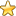Esoteric Wisdom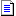# The 16 Binary Permutations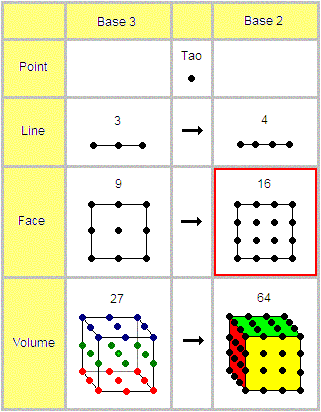(Click on the image map above for easy navigation)

The 16 permutations are the base 2 expansions of the 9 base 3 permutations. They are identical to the 16 geomantic figures although I am sceptical about the concepts traditionally attached to them.

The diagram below shows the permutations in their differentiated configuration. Note that the diagram shows the relative polarity, the bi-elemental nature and the base 10 value for each permutation.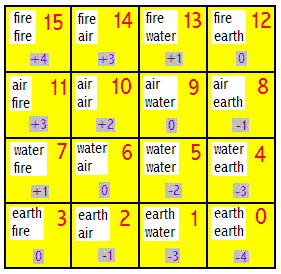This diagram shows the 16 permutations in their undifferentiated configuration: a Magic Square totaling 30 in every direction. Note the diagram which shows the internal structure,ie the linear numerical sequence from 0-15.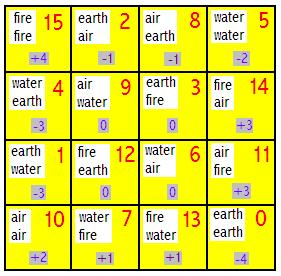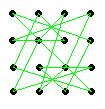The 16 permutations fit exactly apon the features of the tetrahedon.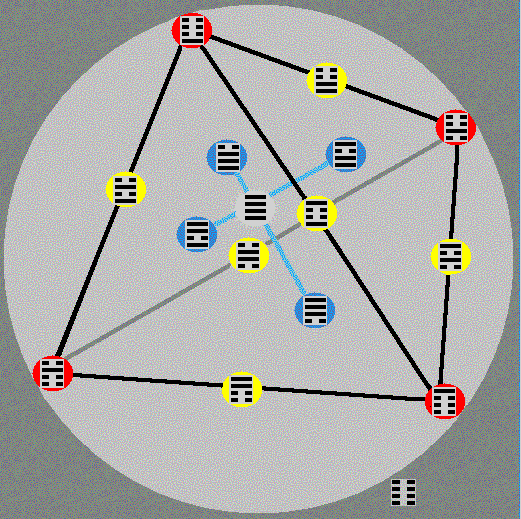In the broadest sense yin and yang can be seen to represent 2 opposites. In the case of the tetrahedon yang represents conciousness and yin represents unconciousness. Therefore:

the 1 permutation comosed entirely of yang lines is positioned centrally.

The 4 permutations with a ratio of 3 yang:1 yin are placed on the four faces which are topographically the nearest features to the center.

The 6 permutations with a ratio of 2 yang:2 yin are placed on the six edges

The 4 permutations with a ratio of 1 yin:2 yang are placed on the four corners which are topographically the furthest featue from the center.

The 1 permutation composed entirely of yin, representing unconciousness is placed hyperthetically on the sphere surounding the tetrahedon.

This 14641 pattern corresponds exactly with the 5th level of Pascal's Triangle

The tetrahedon also forms a perfect 3 dimensional diagram for the 5 Elements and their relationships:
The Tetrahedron and the 5 Elements

You are here: HomeEsoteric Wisdom